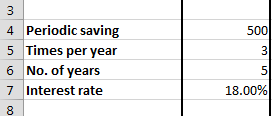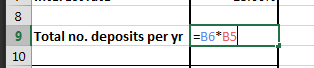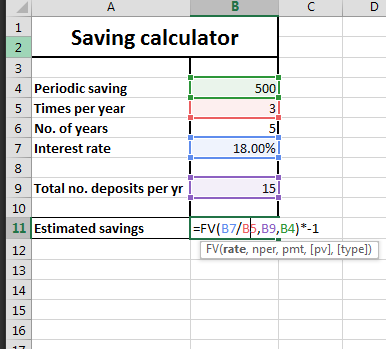### Saving Calculator In Excel

Use Excel application to calculate how much money you will save. Use this free calculator to do that.

1. Open Excel and make four rows which are periodic savings, times per year, no. of years, interest rate, total no. of deposits and estimated savings.2. Enter your data in the cells corresponding the headings: periodic savings, times per year, no. of years and interest rate.3. Calculate total number of deposits. It is simply calculated by multiplying times per year and no. of years.4. Now calculate estimated savings using FV function in excel in the cell corresponding estimated savings. Formula goes as follows:

=FV(interest rate/times per year, total number of deposits, periodic savings)*-1The full formula on Saving Calculator is:

=FV(B7/B5,B9,B4)*-1

## Template

```Further reading: